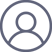周祯祥2021-6-18 16:501、本原函数，算子与递归

01.幺函数I：函数值与自变元的值相同，即Ix=x

03.零函数0：函数值永为零，即0x=0

05.相等性函数eq（x，y）：当x=y时，eq(x,y)值为0，当x≠y时，eq(x,y)值为1。

14.加法+x+y函数的值为x的第y个后继数.

15.减法-x-y函数的值为x的第y个前驱（当xy时，x-y之值为0）。

x+y是个函数，因为它不仅仅包括加法运算+，还包含那个被运算客体xy，算子只是这个函数中的一个转换功能而已。用俗世的一个说法，两个自然数变元xy，或者更细致一点，自然数的两个变元xy给它赋值，那就只是两个自然数的个体而已。它们躺平在那里，如果没有某个力的推动，它会永远躺平在那里，与世无争，自乐自为。但在其中加个加法算子或者其它什么算子，这两个自然数会转换成另一个自然数。两个躺平的自然数，由此就被算子给激活了，它们开始发生了变化，从原来的情形，变成了另外的一种情形。

2、递归函数和原始递归函数

Φ(0，x2x3...xn) = Ψ(x2x3...xn)

Φ(k+1，x2x3...xn) = μ(k，Φ（k，x2x3...xn) （2）5.相等性函数eq（x，y）：当x=y时，eq(x,y)值为0，当x≠y时，eq(x,y)值为1。

γ(x,y)=0, 如果x=y; γ(x,y)=1,如果x≠y。S(x,y)≡($x)[x ≤ Φ(x)∧R(x，y)] T(x,y)≡("x)[x ≤ Φ(x)→R(x，y)]（源文本～似误打，改为等价符号≡） 同样，以下函数Ψ也是递归的 Ψ(x,y)=min x[x ≤ Φ(x)∧R(x，y)] 其中，min xF(x)意味着：那个最小的的数x，对于这个数而言，F(x)成立；并且，如果没有这样的数，则数字为0。 哥德尔用语简洁，命题4实际上是三个支命题，分别为命题4.1，命题4.2与命题4.3。他在文本上已经给出证明的思路，这里按照他的思路给出证明的步骤。 命题4.1. 如果函数Φ(x)与关系R(x，y)是递归的，那么下述关系S也是递归的。 S(x,y)≡($x)[x ≤ Φ(x)∧R(x，y)]

T(x,y)≡("x)[x ≤ Φ(x)→R(x，y)]

Ψ(x,y)=min x[x ≤ Φ(x)∧R(x，y)]

R(χ,η)≡[ρ(χ,η)=0],（源文本～似误打，改为等价符号≡）

Χ(0,η)=0

Χ(n+1,η)=(n+1).α+Χ(n,η).α(α)

α=α[α(ρ(0,η))].α[ρ(n+1,η)].α[Χ(n,η)].～（（R(0,η)）∧R(n+1,η)∧[Χ(n,η）=0].

Ψ(χ,η)=Χ(Φ(χ),η)

S(χ,η)≡R[Ψ(χ,η),η]（源文本～似误打，改为等价符号≡）